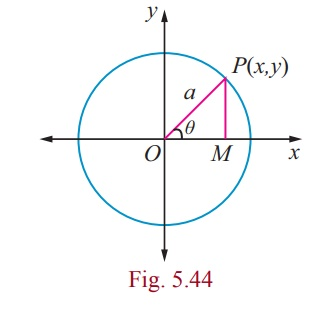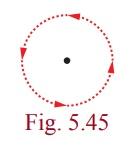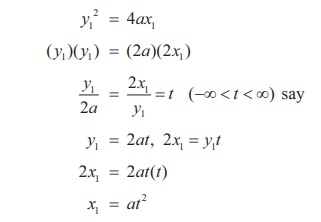Home | | Maths 12th Std | Parametric form of Conics

# Parametric form of Conics

Suppose f (t) and g(t) are functions of 't '. Then the equations x = f (t) and y = g(t) together describe a curve in the plane .

Parametric form of Conics

## 1. Parametric equations

Suppose f (t) and g(t) are functions of 't '. Then the equations x = f (t) and y = g(t) together describe a curve in the plane . In general 't ' is simply an arbitrary variable, called in this case a parameter, and this method of specifying a curve is known as parametric equations. One important interpretation of  't ' is time . In this interpretation, the equations x = f (t) and y = g(t) give the position of an object at time 't ' .

So a parametric equation simply has a third variable, expressing x and y in terms of that third variable as a parameter . A parameter does not always have to be 't ' . Using 't ' is more standard but one can use any other variable.

## (i) Parametric form of the circle x2+ y2 = a2Let P(x, y) be any point on the circle x2 + y2 = a2.

Join OP and let it make an angle θ with x -axis.

Draw PM perpendicular to x -axis. From triangle OPM ,

x = OM = a cosθ

y = MP = a sinθ

Thus the coordinates of any point on the given circle are (a cos θ, a sin θ) and

x = a cosθ , y = a sinθ , 0 ≤ θ ≤ 2π are the parametric equations of the circle x2 + y2 = a2.

Conversely, if x = a cosθ , y = a sinθ , 0 ≤ θ ≤ 2π ,Thus x2 + y2  = a2 yields the equation to circle with centre (0, 0) and radius a units.

Note

(1) x = a cos t, y = a sin t , 0 ≤ t ≤ 2π also represents the same parametric equations of circle x2 + y2 = a2

t increasing in anticlockwise direction.(2) x = a sin t, y = a cos t, 0 ≤ t ≤ 2π also represents the same parametric equations of circle x2 + y2 = a2,

t increasing in clockwise direction.## (ii) Parametric form of the parabola y2 = 4ax

Let P(x1 , y1) be a point on the parabola

y12 = 4ax1Parametric form of y2 = 4ax is x = at2, y = 2at, −∞ < t < ∞ .

Conversely if x = at2 and y = 2at, −∞< t < ∞ , then eliminating 't ' between these equations we get y2 = 4ax .

## (iii) Parametric form of the EllipseLet P be any point on the ellipse. Let the ordinate MP meet the auxiliary circle at Q .Let ACQ = α

CM  = a cosα , MQ = a sin α

and Q(a cosα , a sin α )

Now x -coordinate of P is a cosα . If its y -coordinate is y′, then P(a cosα , y′) lies onHence P is (a cosα , b sin α ) .

The parameter α is called the eccentric angle of the point P . Note that α is the angle which the line CQ makes with the x -axis and not the angle which the line CP makes with it.

Hence the parametric equation of an ellipse is x = a cosθ , y = b sinθ , where θ is the parameter 0 ≤ θ ≤ 2π .

## (iv) Parametric form of the HyperbolaSimilarly, parametric equation of a hyperbola can be derived as x = a secθ , y = b tanθ , where θ  is the parameter.  −π  θ π except θ = ± π/2.

In nutshell the parametric equations of the circle, parabola,ellipse and hyperbola are given in the following table.### Remark

(1) Parametric form represents a family of points on the conic which is the role of a parameter. Further parameter plays the role of a constant and a variable, while cartesian form represents the locus of a point describing the conic. Parameterisation denotes the orientation of the curve.

(2) A parametric representation need not be unique.

(3) Note that using parameterisation reduces the number of variables at least by one.

Study Material, Lecturing Notes, Assignment, Reference, Wiki description explanation, brief detail
12th Mathematics : UNIT 5 : Two Dimensional Analytical Geometry II : Parametric form of Conics |